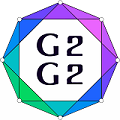#Graphs and Groups, Geometries and GAP (G2G2) Summer School - External Satellite Conference of 8ECM

27 June 2021 to 3 July 2021
Rogla, Slovenia
UTC timezone

## Rigidity of $v_3$-configurations

Not scheduled
20m
Rogla, Slovenia

#### Rogla, Slovenia

Hotel Planja, Rogla 1, 3214 Zreče, Slovenia
Oral presentation

Signe Lundqvist

### Description

A framework of a graph is rigid if no motion of the graph, preserving edge lengths, changes the distance between two vertices. Equivalently, a graph is rigid if it can only move by translation and rotation. A graph that is not rigid is flexible. Under certain conditions on the coordinates of the vertices, we can determine whether or not a planar framework of a graph is rigid by looking at the underlying graph.

A geometric $v_k$-configuration is a collection of $v$ straight lines and $v$ points in the plane such that there are $k$ points on each line and $k$ lines through each points. A geometric $v_2$-configuration is a framework of a graph, so geometric $v_k$-configurations generalise frameworks of graphs.

In this talk we will consider the rigidity properties of $v_k$-configurations. A geometric $v_k$-configuration is rigid if the only motions of it, preserving point-line incidences and distances between collinear points, are translation and rotation. A geometric $v_k$-configuration that is not rigid is flexible.